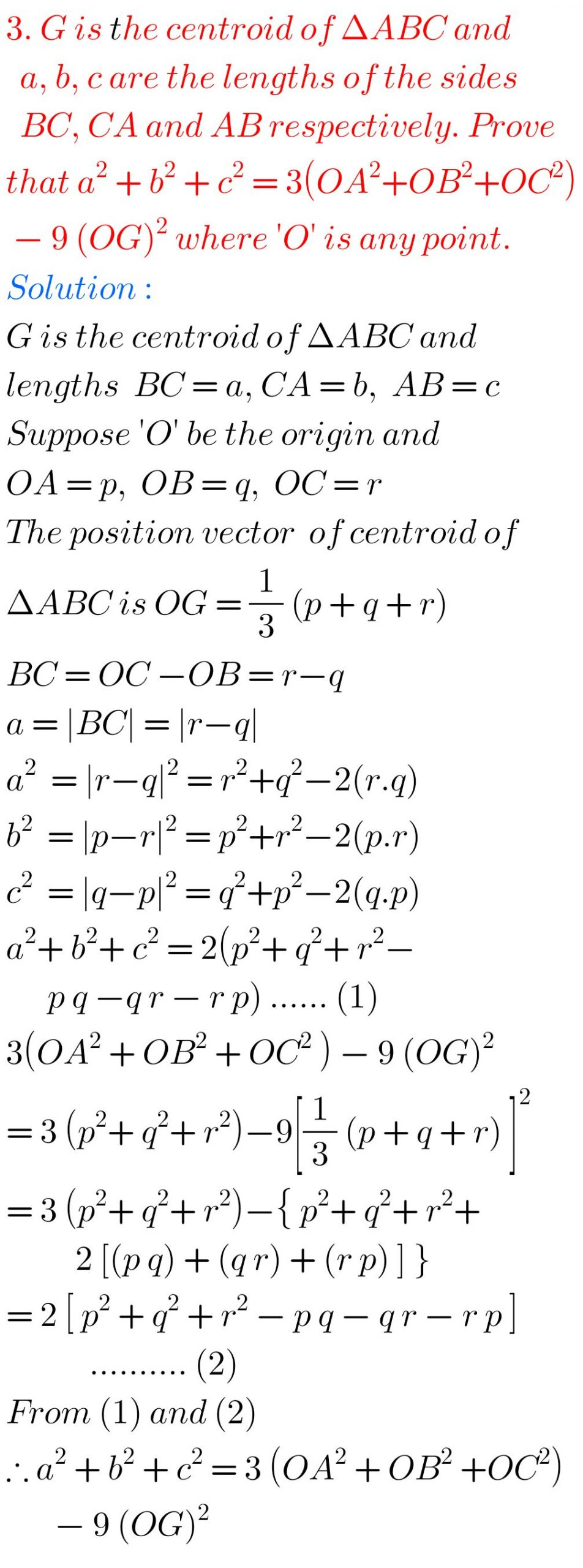## Intermediate Maths Solutions for Exercise 5(a) Product of Vectors

Inter Solutions for Maths 1A Exercise 5(a) Product of Vectors Intermediate mathematics IA Exercise 5(a) Product of Vectors textbook solutions are given. These solutions are very easy to understand. Please study the textbook lesson Product of Vectors very well. Then observe the example problems and solutions given in the text book. Try them. Observe the …